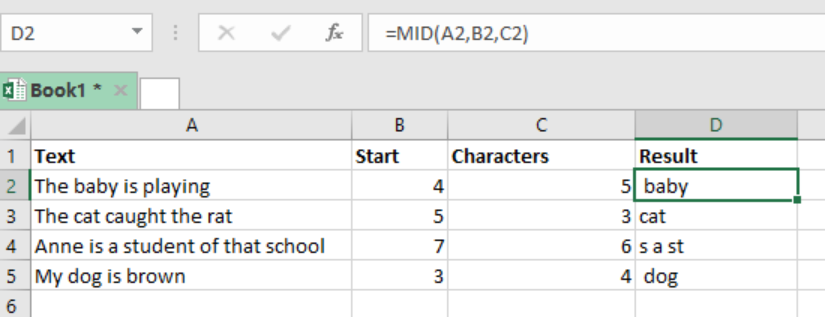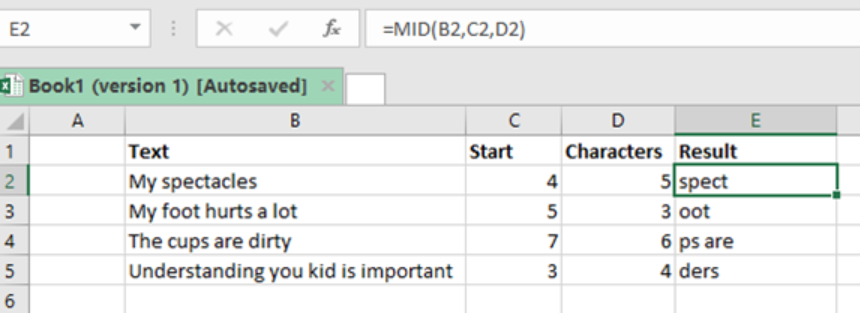Get instant live expert help with Excel or Google Sheets“My Excelchat expert helped me in less than 20 minutes, saving me what would have been 5 hours of work!”

#### Post your problem and you’ll get expert help in seconds.

Your message must be at least 40 characters
Our professional experts are available now. Your privacy is guaranteed.

# Excel MID Function

We can use the Excel MID function to extract given characters from the middle of a text string. While this might seem challenging to some people, this post provides a clear guide on how extract the middle characters of a supplied text string using the excel MID function.Figure 1: Finding md characters in a text string

## Syntax of the formula

`=MID (text, start_number, num_chars)`

Where;

• Text- refers to the text string we want to extract from.
• Start_num- refers to the location of the first character to extract.
• Num_chars– refers to the number of characters to extract.

## Understanding the Excel MID function

As already stated, the Excel MID function is necessary if we are to extract characters from a given text string. This function works by;

• It returns the number of characters which you have specified from the text string.
• It starts from the “start_num” and goes through to “start_num + num_chars”
• Used when we want to extract text from inside a text string, based on a given location and length.
• To find the location if we don’t know, we can use the FIND or SEARCH functions to locate the “start_num”.

## ExampleFigure 2: Using Excel MID function to find mid characters in a text string

Step 1: Prepare the data sheet with columns for “text string”, “start”, “characters” and “result”.

Step 2: In cell E2, enter the formula; i.e.` =MID (B2,C2,D2)`

Step 3: Press Enter

Step 4: Copy the formula across the other cells

## Instant Connection to an Expert through our Excelchat Service

Most of the time, the problem you will need to solve will be more complex than a simple application of a formula or function. If you want to save hours of research and frustration, try our live Excelchat service! Our Excel Experts are available 24/7 to answer any Excel question you may have. We guarantee a connection within 30 seconds and a customized solution within 20 minutes.

### Did this post not answer your question? Get a solution from connecting with the expert.Another blog reader asked this question today on Excelchat:
Solution examplesI need a formula .... i need to decrease the premium an employee pays by 10% each year for ages 65-75. So when they are 65 it decreases 10%, age 66 it decreases 20% and so on. I tried =IF(OR(\$D2+1<65,\$D2+1=66,\$D2+2=77, ...... ),J2,J2-(J2*0.1),J2(J2*0.2) .... ) D2 being the current age of the employee, with each column being year 1, year 2, etc. However, excel will not allow me to do to IF D2+1=66, til age 75. It's too long. I know there has to be a better way! HELP!
Solved by F. F. in 60 minsCan you re edit this formula for me =IFERROR(IF(LEN(MID("RHS",1,FIND("RHS",B4,1)))=1,LEFT(B4,3),MID("RHS",1,FIND("RHS",B4,1))),MID("LHS",1,FIND("LHS",B4,1)))
Solved by S. L. in 40 minsI have a column where each cell has letters and numbers. The numbers would range from 10 through 18. I would like to find a formula where I can search the cell for any one of the numbers and return a value from a different cell if it finds one. I currently search for a specific number and the formula works but only for that particular number. The formula I currently have is: =IF(ISNUMBER(SEARCH("16",F10)),G10,"") This does work if it finds the value of 16 in the (F10)cell and then places the value from cell "G10" as the result. If it doesn't find the numbers, it will leave the resultant cell blank.
Solved by O. H. in 60 minsIf I have a sheet that pulls data from another sheet. How can I compare if the right data is filled in, after the fact?
Solved by Z. Y. in 43 minsI have text and number in multiple cells (Ex: Cell 1: 4S, Cell 2: 3.5A, Cell 3: 8O) and want to sum the number values only (Ex: 4 + 3.5 + 8). How can I do this?
Solved by O. A. in 60 mins## Subscribe to Excelchat.coAnother blog reader asked this question today on Excelchat: